#Function Repository Resource:

# Spirograph

Plot a spirograph

Contributed by: Wolfram Staff
 ResourceFunction["Spirograph"][f,φ] plots a spirograph for function f with variable φ. ResourceFunction["Spirograph"][f,φ,φf] plots a spirograph from 0 to φf.

## Details and Options

Imagine a wheel rolling around a second wheel that is rolling around another wheel and so on. A point on the rim of the outermost wheel will trace an interesting curve in the plane. Let the radius and angular frequency of the jth wheel be rj and nj. The point will be at the position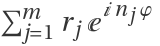in the complex φ-plane. The function ResourceFunction["Spirograph"] generates a picture of the path of the point.
Assume that there is a closed parametrized curve in the plane. Find the points pk on the curve corresponding to a division of the parameter domain into m d parts, where m and d are positive integers. The d m-sided Maurer polygons (1989) are formed by joining the points pk,pk+d,pk+2d,,pk+(m-1)d,pk, for k=1,2,,d.
Option "MaurerPolygons" default value for m and d is {100,400} when set to True.

## Examples

### Basic Examples

Plot a spirograph:

 In:=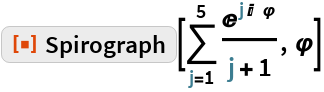Out=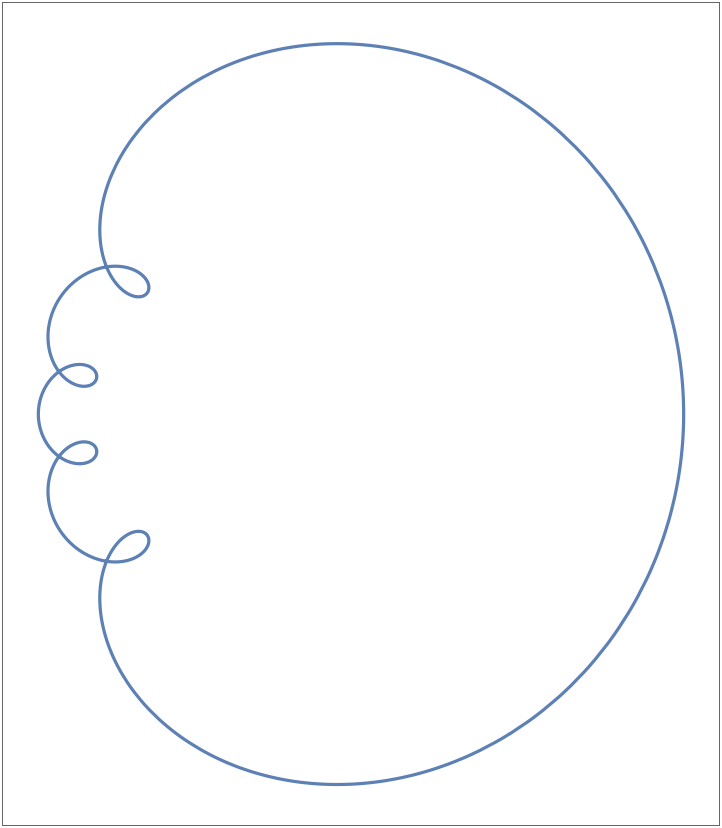A more complicated spirograph:

 In:=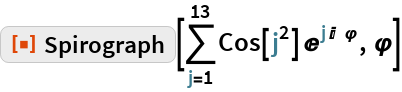Out=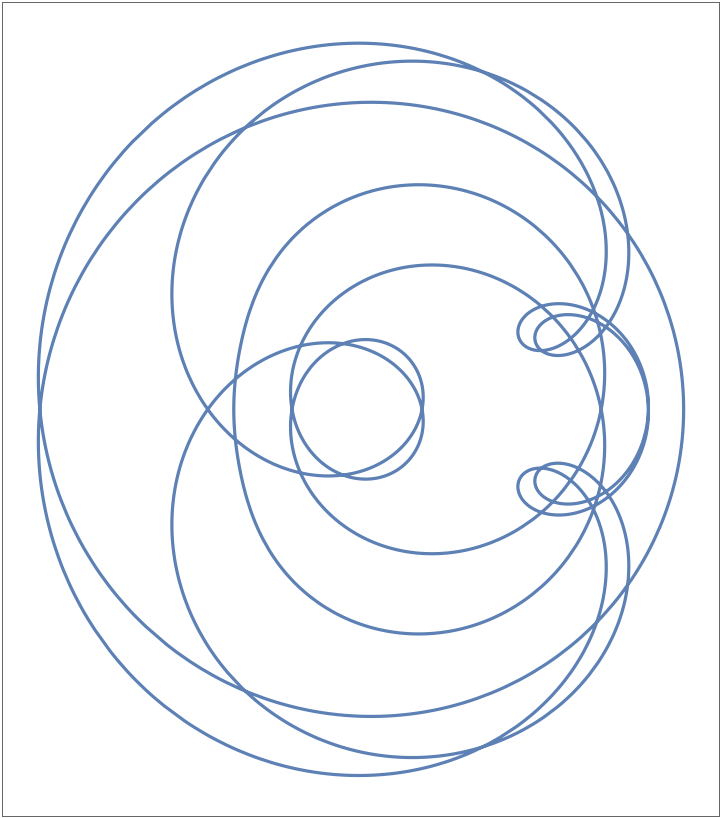Half of the path of the spirograph:

 In:=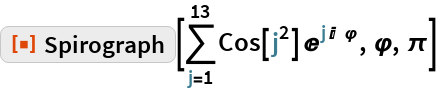Out=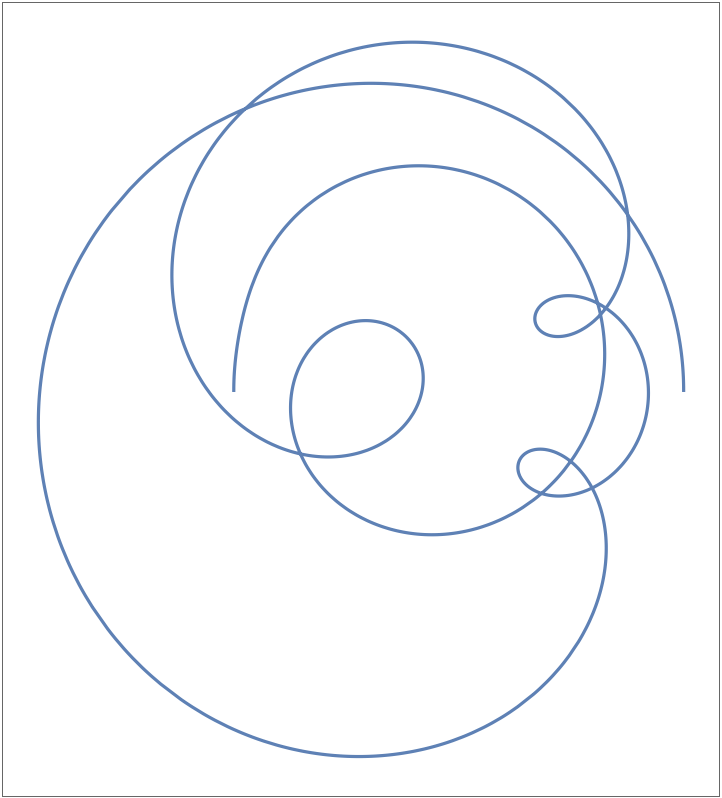Convert the curve to a polygon:

 In:=Out=Spirographs with random values:

 In:=Out=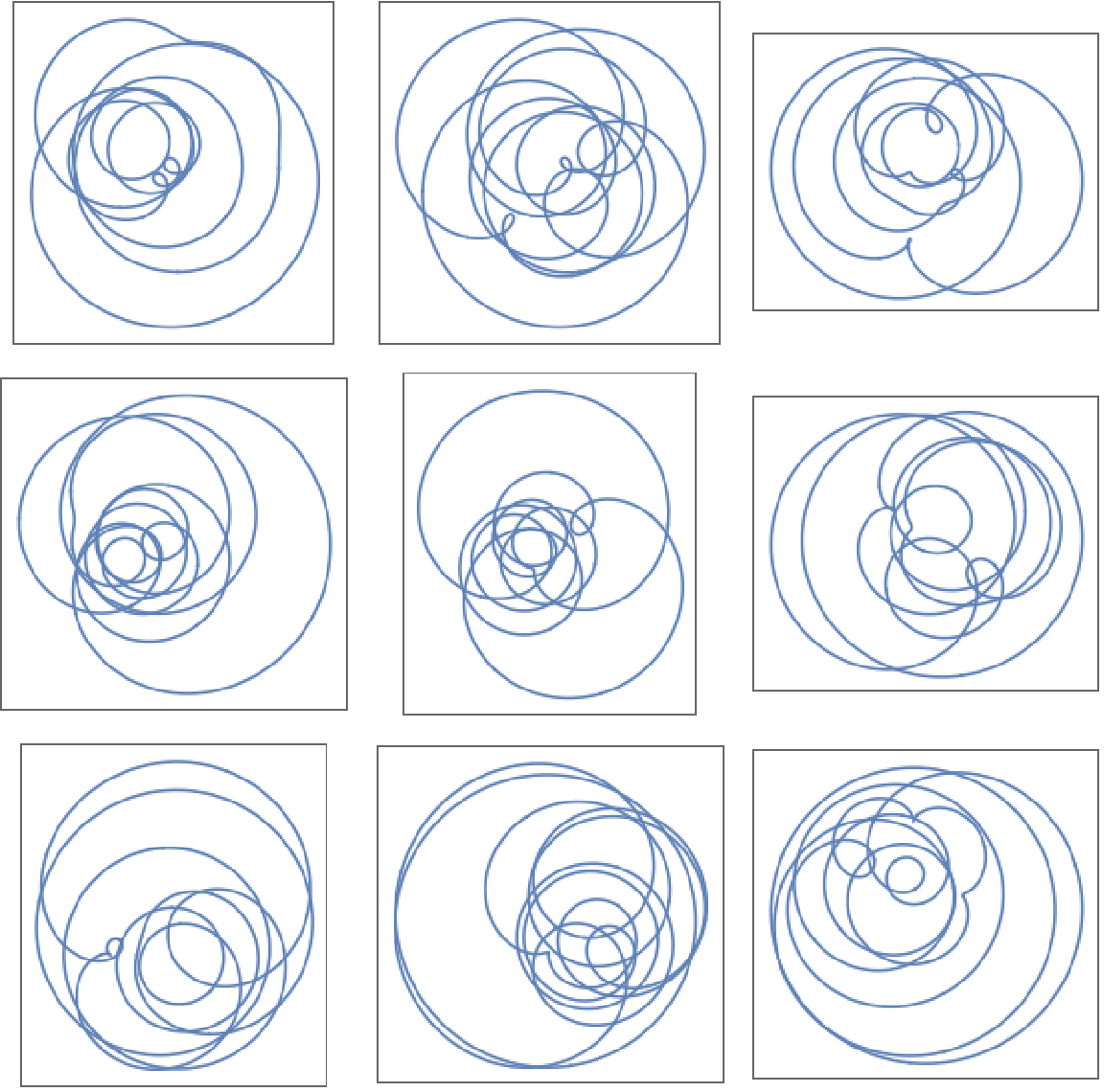### Options

#### ColorFunction

Choose a ColorFunction:

 In:=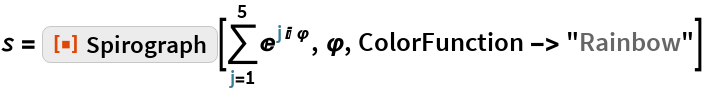Out=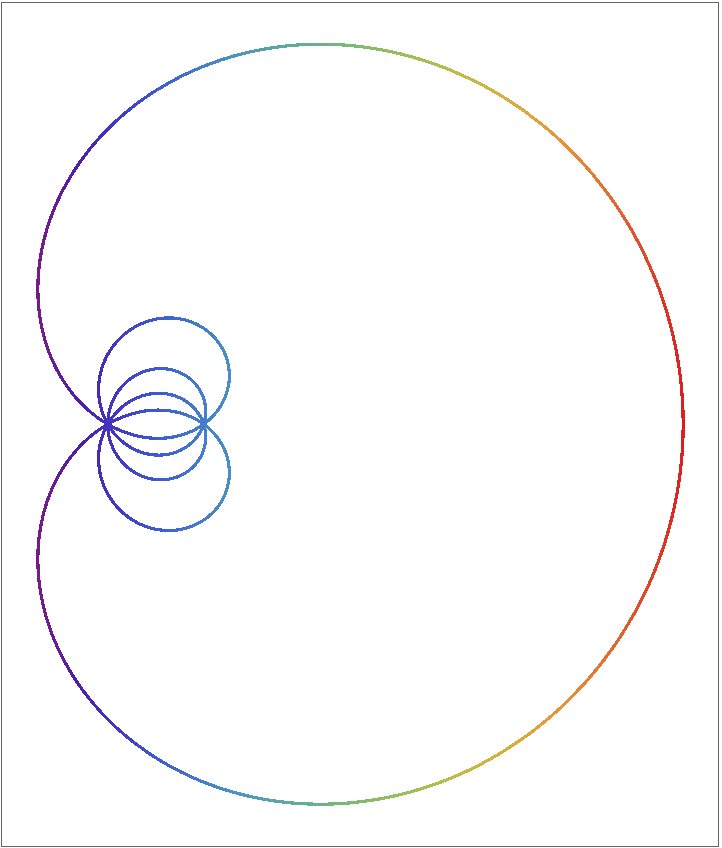#### MaurerPolygons

Set explicit values for “MaurerPolygons”:

 In:=Out=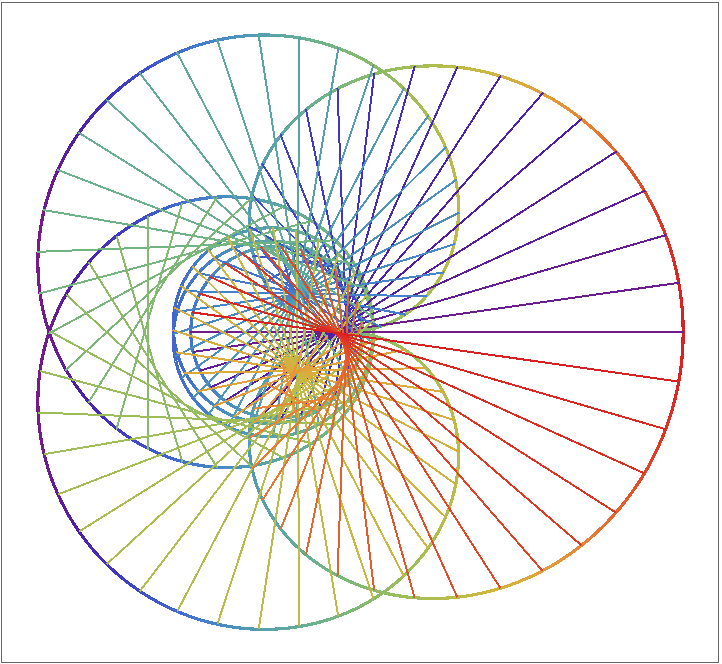Default values for “MaurerPolygons”:

 In:=Out=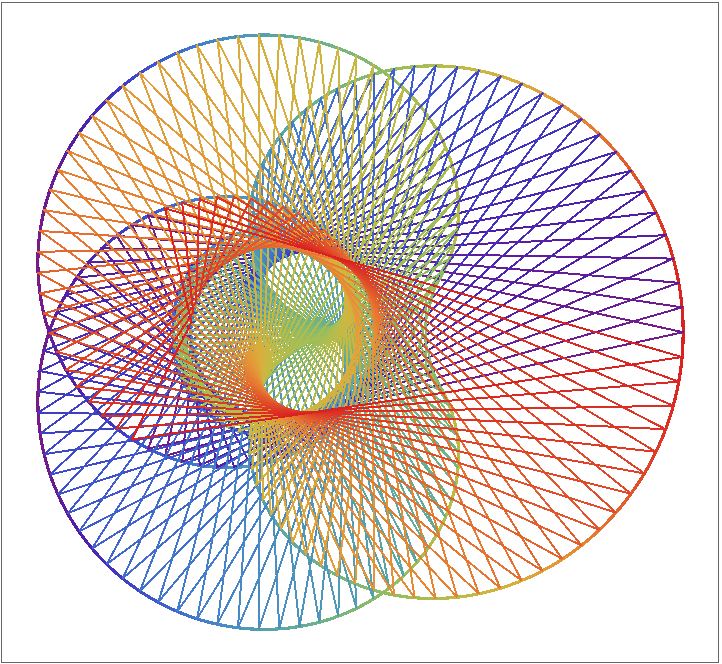#### ShowCircles

Show the “wheels” that generate the spirograph:

 In:=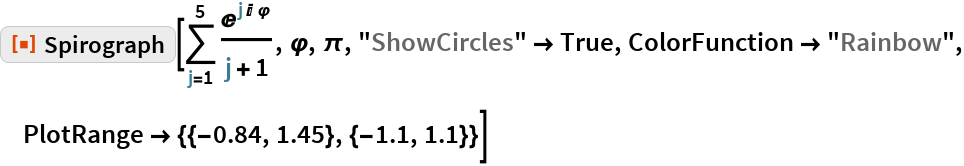Out=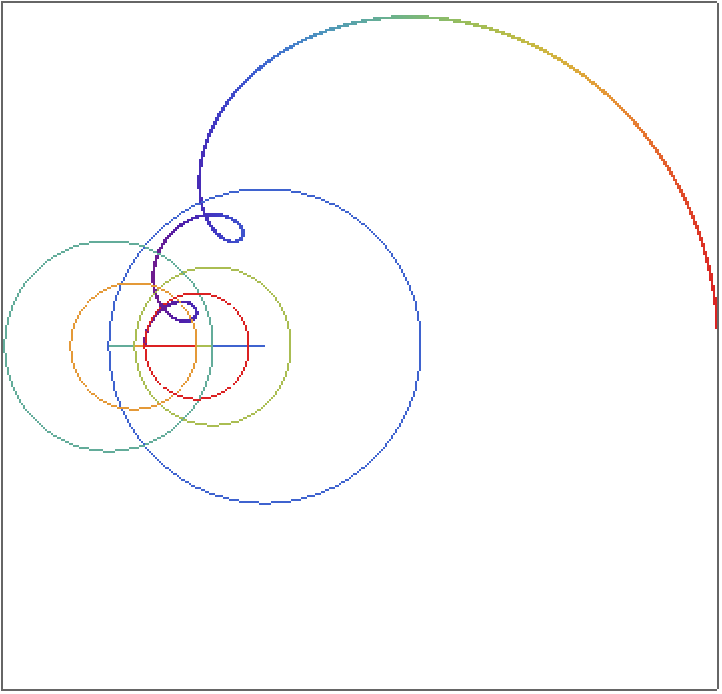Make an animation:

 In:=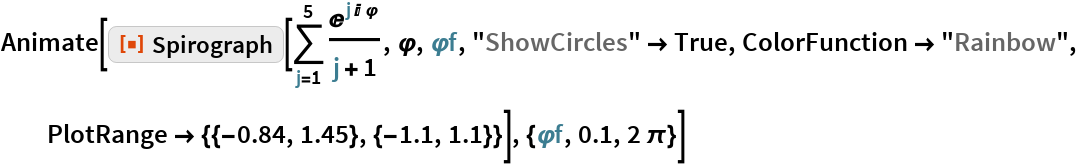Out=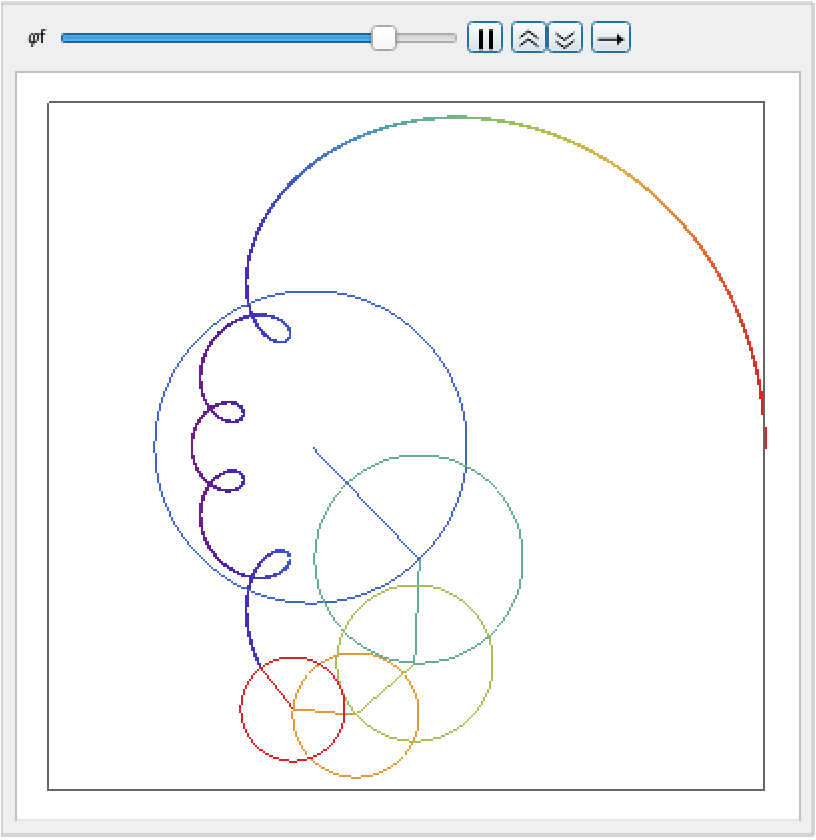### Applications

Spirographs had an important application in the 1940s, when they were used for the "manual" solution of polynomial equations of higher degree. To understand this, consider the following polynomial poly in the variable z:

 In:=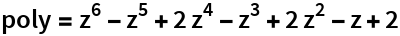Out=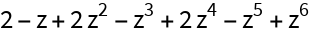Replace the variable z by its polar form, r ⅇⅈ φ , where r and φ are the absolute value and phase or argument of z:

 In:=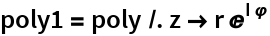Out=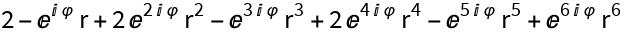Show the spirographs for a range of values of r:

 In:=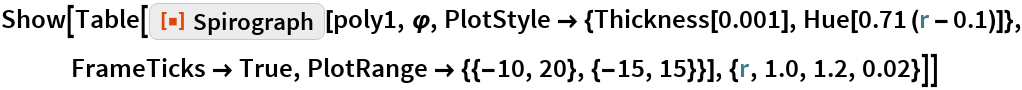Out=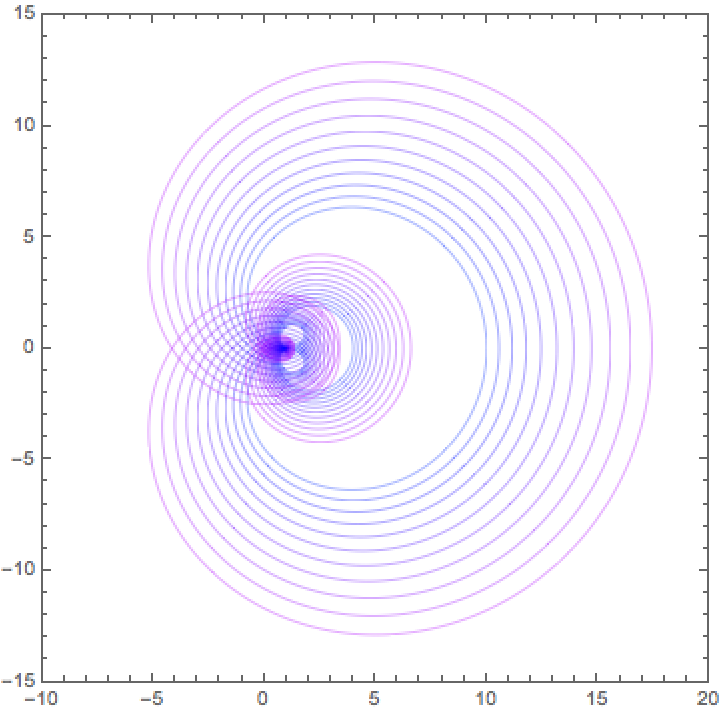Zoom to a neighborhood of the origin:

 In:=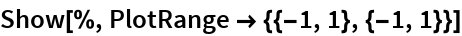Out=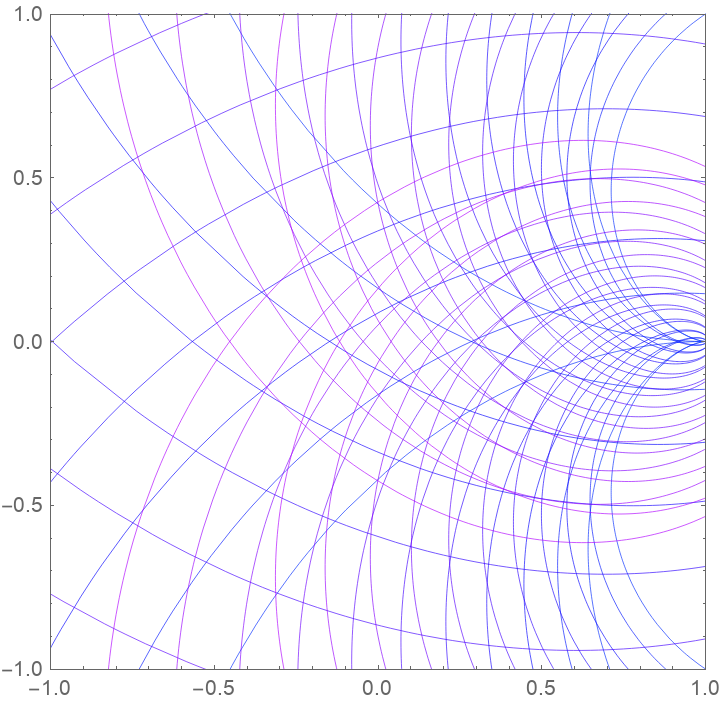Here is an even closer look:

 In:=Out=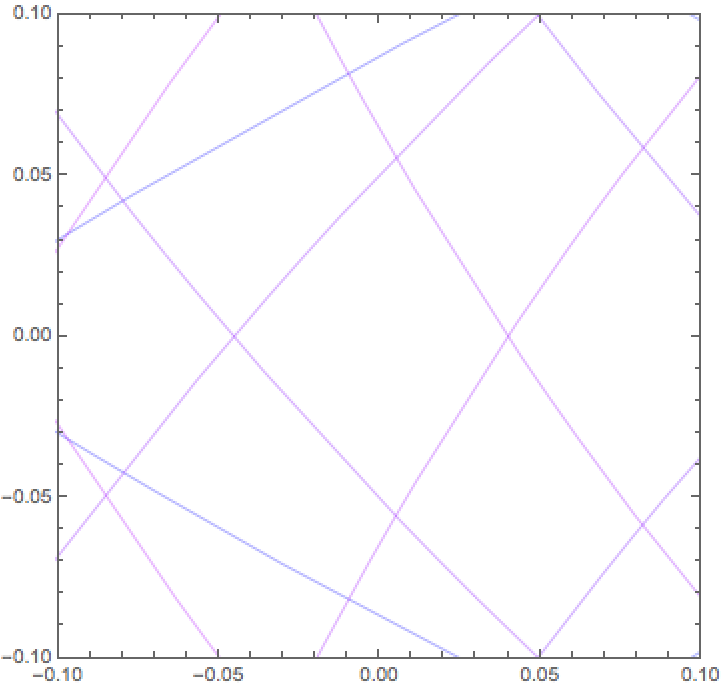These are absolute values and arguments of the zeros of the polynomial:

 In:=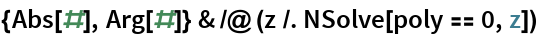Out=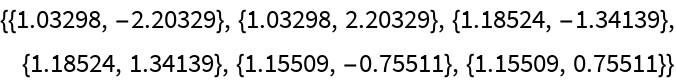The spirograph curves corresponding to these absolute values all go through the origin:

 In:=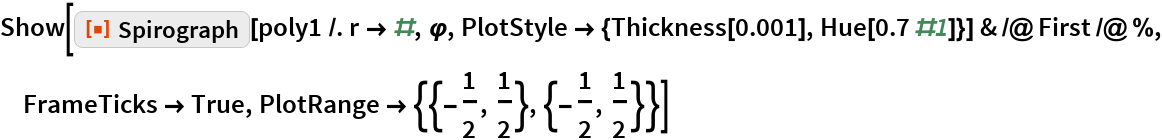Out=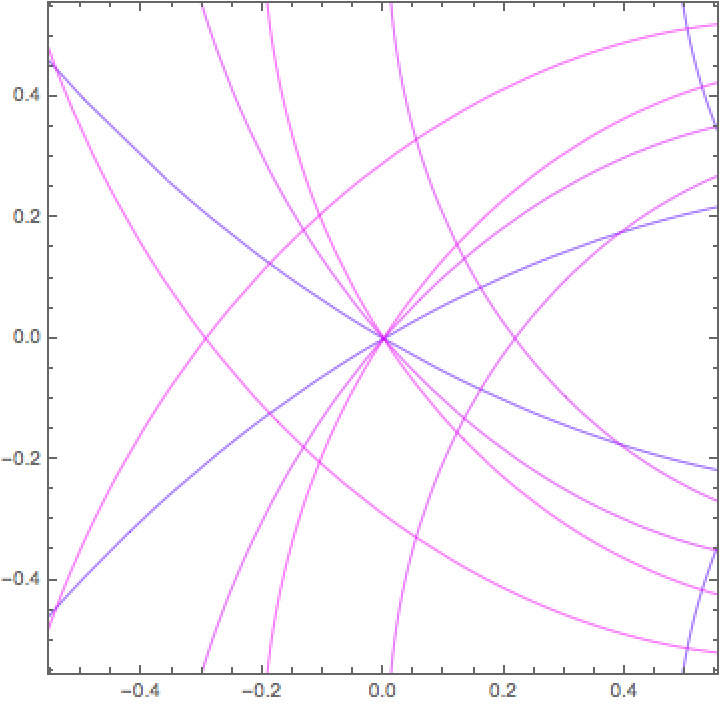An analog circuit was used to vary r and the corresponding spirographs were monitored using an oscilloscope. Further analysis to determine φ led to approximations of the zeros of the polynomial.

## Requirements

Wolfram Language 11.3 (March 2018) or above